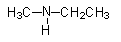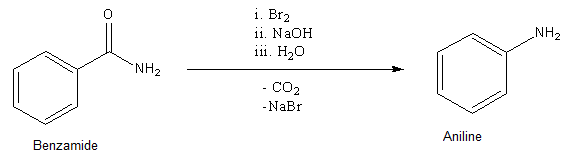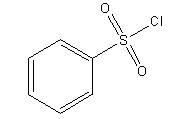QuestionAnswers

# (a)(i) Explain Hoffman bromamide degradation for the preparation of Aniline.(ii) Give the IUPAC name of(b) What is Hinsberg reagent ? Between $C{H_3}N{H_2}$ and ${C_6}{H_5}N{H_2}$ which is more basic ?Hint: Hoffman bromamide degradation converts $- CON{H_2}$ functional group into $- N{H_2}$. Hinsberg reagent is used in separation of mixtures of amines. As N-atoms get more nucleophilic, its basicity also increases.

Complete step by step solution:

(a) (i)
- Hoffmann bromamide degradation is useful in production of Aniline as it converts the$- CON{H_2}$ functional group into the$- N{H_2}$group. So, we need to take a starting material that has the$- CON{H_2}$ group and after getting converted into $- N{H_2}$ group, the molecule obtained is Aniline. So, in order to obtain Aniline as the final product, we require Benzamide as a starting material. The reaction is as shown below.So, in this way, we can easily convert Benzamide to Aniline.
Now, we will try to assign IUPAC names to this compound.

ii)Amines can be named in two ways. We can use the amino word as a prefix and amine word can be used as a suffix as well. Both names are legal according to IUPAC nomenclature.
- First identify the longest alkyl chain, which is of two carbons. There is a substitution of methyl groups on the N-atom. But we will need to specify the place of attachment of the methyl group as N-methyl.
So, its IUPAC name will be N-methylethanamine.
If we use amino prefix, then it will be named as N-methylaminoethane.

(b) Hinsberg reagent is Benzenesulfonyl chloride. Its structure is shown below.- It is used in separation of mixture of primary, secondary and tertiary amines. Here, atom of amines attack electrophilic S-atoms and undergo reaction.
- Basicity of amines depends upon the ability of atom to donate electrons. Let’s compare basicity of
$C{H_3}N{H_2}$ and ${C_6}{H_5}N{H_2}$.
- In Methylamine, $- C{H_3}$ is an electron donating group, so it will push electron density towards the N-atom.
- In case of Aniline, N-atom is directly bonded to the benzene ring. So, N-atom is also involved in resonance structures and electron density is not always concentrated on N-atom, so it has comparatively less efficiency to donate electrons than Methyl amine. Also, aromatic rings act as an electron withdrawing group, so that will also reduce electron density on the N-atom.
So, Methyl amine is more basic than Aniline
- The order of basicity of amines are as follows.
Primary < Secondary < Tertiary
- Hoffman bromamide degradation can be categorized as a rearrangement reaction.

Note: While naming a compound, make sure that you choose the correct parent alkyl chain, otherwise the name will be wrong. Note that Hoffman bromamide degradation involves rearrangement of atoms, which is an exception.

NCERT Book for Class 12 Chemistry PDF in HindiPreparation of Standard Solution of Oxalic AcidPreparation of Hydrogen and Its UsesNCERT Book for Class 11 Chemistry PDF in HindiNCERT Book Class 12 Chemistry PDFNCERT Book for Class 12 Accountancy PDFI Love My Family EssayNCERT Books for Class 12 PDF in HindiImportant Questions for CBSE Class 12 ChemistryImportant Questions for CBSE Class 12 Chemistry Chapter 5 - Surface ChemistryImportant Questions for CBSE Class 12 Chemistry Chapter 1 - The Solid StateImportant Questions for CBSE Class 12 Chemistry Chapter 16 - Chemistry in Everyday lifeImportant Questions for CBSE Class 12 Chemistry Chapter 3 - ElectrochemistryImportant Questions for CBSE Class 12 Chemistry Chapter 7 - The p-Block ElementsImportant Questions for CBSE Class 12 Chemistry Chapter 13 - AminesImportant Questions for CBSE Class 12 Chemistry Chapter 14 - BiomoleculesImportant Questions for CBSE Class 12 Chemistry Chapter 2 - SolutionsImportant Questions for CBSE Class 11 Chemistry Chapter 12 - Organic Chemistry - Some Basic Principles and TechniquesChemistry Question Paper for CBSE Class 12Chemistry Question Paper for CBSE Class 12 - 2013Chemistry Question Paper for CBSE Class 12 - 2015CBSE Class 12 Chemistry Question Paper 2020Previous Year Question Paper for CBSE Class 12 Chemistry - 2014CBSE Class 12 Chemistry Question Paper 2019 - Free PDFCBSE Class 12 Chemistry Question Paper 2017 - Free PDFChemistry Question Paper for CBSE Class 12 - 2016 Set 1 EChemistry Question Paper for CBSE Class 12 - 2016 Set 1 SCBSE Class 12 Chemistry Question Paper 2018 - Free PDFSurface Chemistry NCERT Solutions - Class 12 ChemistryNCERT Solutions for Class 12 ChemistryNCERT Solutions for Class 12 ChemistryNCERT Solutions for Class 12 Chemistry Chapter 5 Surface Chemistry in HindiNCERT Exemplar for Class 12 Chemistry Chapter-12 (Book Solutions)NCERT Solutions for Class 12 Chemistry Chapter 2NCERT Solutions for Class 12 Chemistry Chapter 1NCERT Solutions for Class 12 Chemistry Chapter 15NCERT Solutions for Class 12 Chemistry Chapter 14NCERT Solutions for Class 12 Chemistry Chapter 6